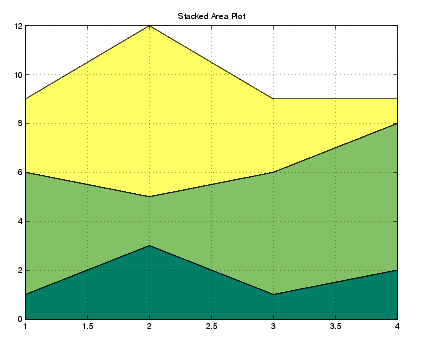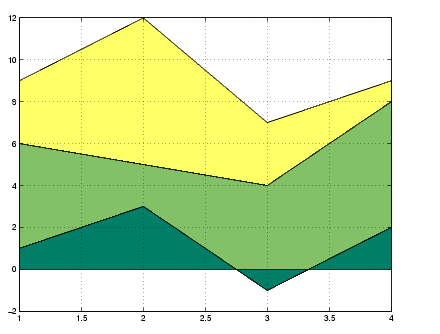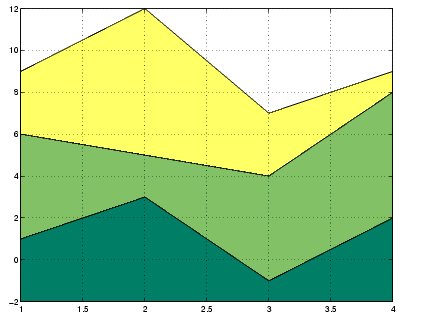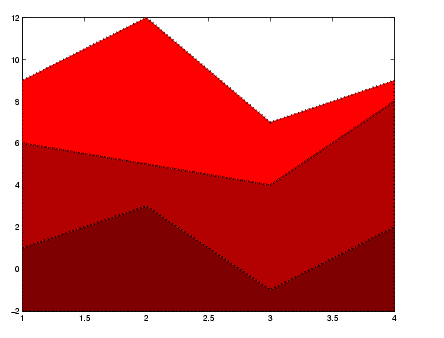MATLAB Function Referencearea

Filled area 2-D plot

Syntax

• ```area(Y)
area(X,Y)
area(...,basevalue)
area(...,'`PropertyName`',PropertyValue,...)
area(axes_handle,...)
h = area(...)
area('v6',...)
```

Description

An area graph displays elements in `Y` as one or more curves and fills the area beneath each curve. When `Y` is a matrix, the curves are stacked showing the relative contribution of each row element to the total height of the curve at each x interval.

```area(Y) ``` plots the vector `Y` or the sum of each column in matrix `Y`. The x-axis automatically scales to `1:size(Y,1)`.

```area(X,Y) ``` For vectors `X` and `Y`, `area(X,Y)` is the same as `plot(X,Y)` except that the area between `0` and `Y` is filled. When `Y` is a matrix, `area(X,Y)` plots the columns of `Y` as filled areas. For each `X`, the net result is the sum of corresponding values from the columns of `Y`.

If `X` is a vector, `length(X)` must equal `length(Y)` and `X` must be monotonic. If `X` is a matrix, `size(X)` must equal `size(Y)` and each column of `X` must be monotonic. To make a vector or matrix monotonic, use `sort`.

```area(...,basevalue) ``` specifies the base value for the area fill. The default `basevalue` is `0`. See the `BaseValue` property for more information.

```area(...,'PropertyName',PropertyValue,...) ``` specifies property name and property value pairs for the patch graphics object created by `area`.

```area(axes_handles,...) ``` plots into the axes with handle `axes_handle` instead of the current axes (`gca`).

```h = area(...) ``` returns handles of areaseries graphics objects.

Backward Compatible Version

```hpatches = area('v6',...) ``` returns the handles of patch objects instead of areaseries objects for compatibility with MATLAB 6.5 and earlier. See patch object properties for a discussion of the properties you can set to control the appearance of these area graphs.

See Plot Objects and Backward Compatibility for more information.

Areaseries Objects

Creating an area graph of an m-by-n matrix creates n areaseries objects (i.e., one per column), whereas a 1-by-n vector creates one area object.

Note that some areaseries object properties that you set on an individual areaseries object set the value for all areaseries objects in the graph. See the property descriptions for information on specific properties.

Examples

Stacked Area Graph

This example plots the data in the variable `Y` as an area graph. Each subsequent column of `Y` is stacked on top of the previous data. Note that the figure colormap controls the coloring of the individual areas. You can explicitly set the color of an area using the `EdgeColor` and `FaceColor` properties.

• ```Y = [1, 5, 3;
3, 2, 7;
1, 5, 3;
2, 6, 1];
area(Y)
grid on
colormap summer
set(gca,'Layer','top')
title 'Stacked Area Plot'```

Adjusting the Base Value

The area function uses a y-axis value of 0 as the base of the filled areas. You can change this value by setting the area `BaseValue` property. For example, negate one of the values of Y from the previous example and replot the data.

• ```Y(3,1) = -1; ```% Was 1
```h = area(Y);
set(gca,'Layer','top')
grid on
colormap summer
```

The area graph now looks like this:Adjusting the `BaseValue` property improves the appearance of the graph:

• ```set(h,'BaseValue',-2)
```

Note that setting the `BaseValue` property on one areaseries object sets the values of all objects.Specifying Colors and Line Styles

You can specify the colors of the filled areas and the type of lines used to separate them.

• ```h = area(Y,-2); ```% Set BaseValue via argument
```set(h(1),'FaceColor',[.5 0 0])
set(h(2),'FaceColor',[.7 0 0])
set(h(3),'FaceColor',[1 0 0])
set(h,'LineStyle',':','LineWidth',2) ```% Set all to same value
``````

See Also

`bar`, `plot`, `sort`

Area, Bar, and Pie Plots for related functions

Area Graphs for more examples

Areaseries Properties for property descriptionsany Areaseries Properties© 1994-2005 The MathWorks, Inc.